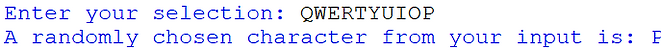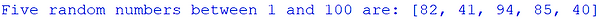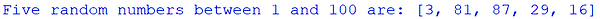## Importing

This section looks at additional commands that you can import and use from Python’s code library.

A library is a collection of different coding sequences that automatically come with Python but are separate from the main file. They can be imported (brought in) to your program by using the import command at the start of your program.

Imagine Python’s library to be similar to an actual library. There are different sections in a real library (such as History, Geography, Reference) and different sections in Python’s library (such as random or time). Each real library has many individual books in each section, just like Python.

For example, below we want to open up Python’s code library, go to the section called random and import just the command called randint.

This is more efficient than just importing the whole random section.

## Random Numbers

To generate random numbers, first import the randint command section from Python’s random code library on the first line of the program:The randint command stands for random integer. In brackets, state the number range to randomly choose from.

The random value must be saved into a variable.Above is a valid random number generation line but it needs the import command and a print line to be a complete program:The range in the brackets of a randint command does not have to be fixed values and could be replaced by variables.

Below is a program where the user selects the upper and lower values of the range:1. Generate a random number between 1 and 5.

2. Ask the user to enter a number between 1 and 5.

3. If the user’s number equals the random number then print “CORRECT!” otherwise print “INCORRECT!”

Example solution:## Random Characters

Rather than just numbers, we can also randomly generate characters from a specified range by using the choice command.

Just like before, you need to import the command from the random library and specify the range in brackets.

Below is a program that randomly chooses a letter of the alphabet:The range of characters to choose from does not have to be solely letters; you could use numbers or punctuation.

Also, the range does not have to be fixed either. Characters can be selected from a variable value instead, such as below:1. Generate a random sample from “aeiou”.

2. Ask the user to input a vowel (make sure they use lowercase letters too).

3. If the user’s vowel matches up with the random sample then output an appropriate response.

4. Print a different response if they do not match up.

Example solution:## Random Sample

To choose more than one value from a set of data, use the sample command.

After you have written this command you need to specify the list of values to sample from and how many values you want to choose:Be careful with your brackets.

The string values must be within speech marks and separated by commas and enclosed within square brackets.

After the list of string values, you need another comma and then a value to denote how many values to select.

Below is a program that selects three values from a list of strings and outputs them. Remember to include the import line at the start for every program using a random command.You can also use the sample command to choose several integers from a given range.

By implementing the range command too, you don’t need to individually write out each value like the string example above.Your aunt is going to have a baby boy.

Create a program that randomly selects 3 male names from a list of 10 possible names.

Example solution:Embedded Python Editor